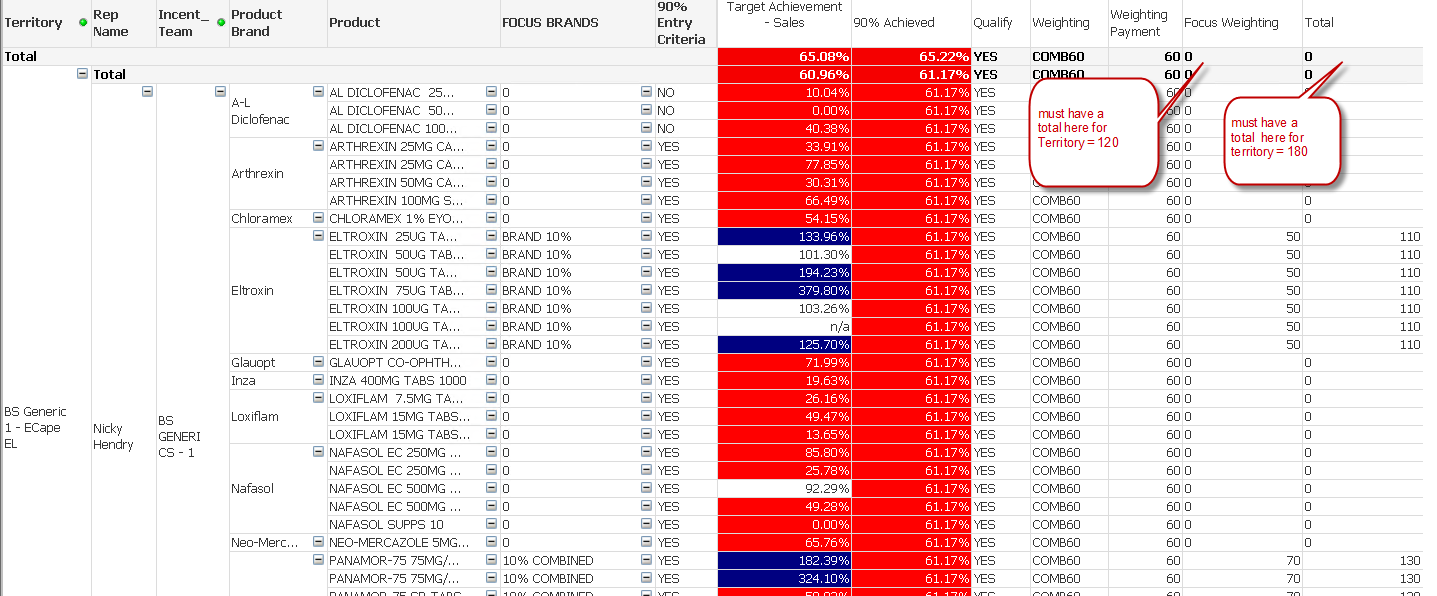# QlikView App Development

Discussion Board for collaboration related to QlikView App Development.

Highlighted
Contributor

## Subtotal in pivot issue

Hi,

I have a subtotal issue, and this cause my [Total] column also not to calculate.

I have a min and max perc that indicate what the rep incentive must be for that line in column [Focus Weighting] – this is a fixed amount. If perc is not in this min and max this value must be 0.

The payout is on a brand level and not on a pack level – meaning you get ‘brand1’ under this you get ‘brand1 pack 30’s’ and ‘brand1 pack 40’s’

The reps work in a Territory that is my first dimension. In properties/presentation I did a partial sum on Territory and Rep Name dimensions where I ticked the checkbox.

I read allot of posts on Aggr on subtotals and tried implementing this, but it also did not work.

In picture the [Focus Weighting] for this Territory must be 120 and the Total for Territory must be 180

I would really appreciate help on these subtotals and total.

my Expression for  [Focus Weighting]  column

if (upper([Qualify])='YES',

if (upper([FOCUS BRANDS])='BRAND 10%',

if(num([90% Achieved], '#,##0%') >= num('0.6100', '#,##0%') and num([90% Achieved], '#,##0%') <= num('0.6200', '#,##0%'),sum (DISTINCT num('50', '#,##0')),

if(num([90% Achieved], '#,##0%') >= num('0.6200', '#,##0%') and num([90% Achieved], '#,##0%') <= num('0.6300', '#,##0%'),sum (DISTINCT num('60', '#,##0'))

,'0')),

if (upper([FOCUS BRANDS])='10% COMBINED',

if(num([90% Achieved], '#,##0%') >= num('0.6100', '#,##0%') and num([90% Achieved], '#,##0%') <= num('0.6200', '#,##0%'), sum (DISTINCT num('70', '#,##0')),

if(num([90% Achieved], '#,##0%') >= num('0.6200', '#,##0%') and num([90% Achieved], '#,##0%') <= num('0.6300', '#,##0%'), sum (DISTINCT num('80', '#,##0')),

,'0'))

)

),'Dont Qualify')thank so much

regards

Louw

Valued Contributor II

## Re: Subtotal in pivot issue

Hi,

maybe you have used for grand total of your expression :

'total of expression'

but the expression can't be evaluated like this because of if (there is not an operator like sum)

so try to change by 'sum of rows' to have grand total

regards